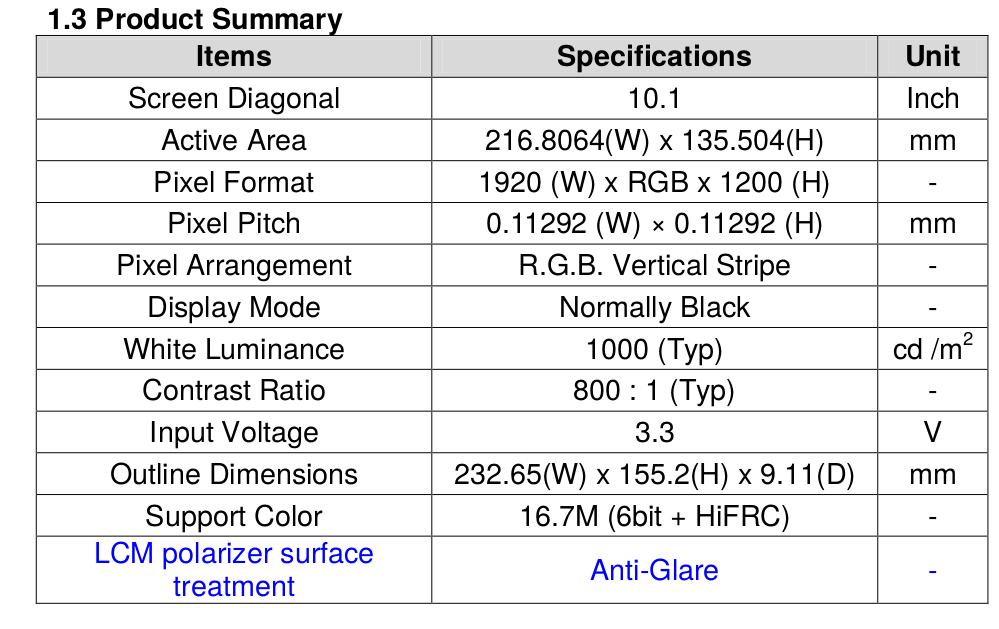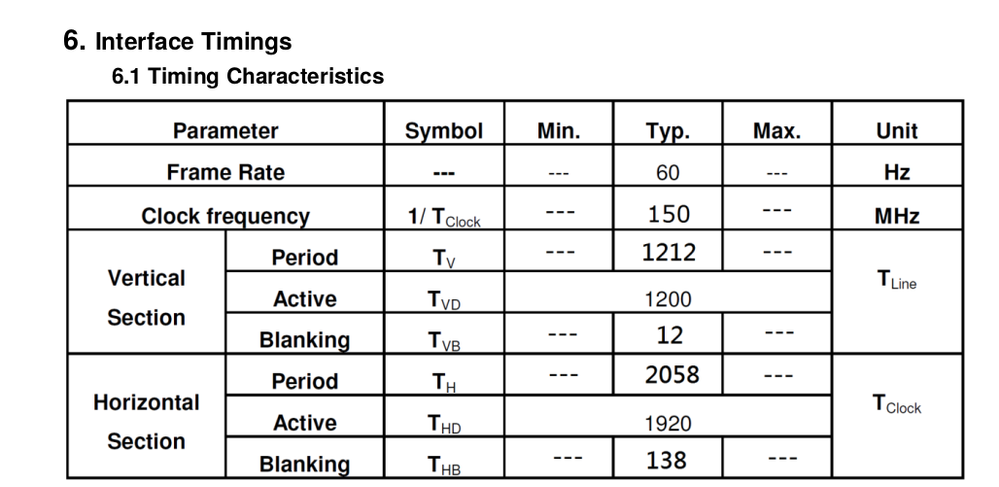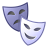## LVDS on IMX6 Linux split-mode

cancel
Showing results for
Did you mean:

## LVDS on IMX6 Linux split-mode

633 ViewsContributor III

I'm try to working with an APALIS from TORADEX (IMX6 quad) to work with an LVDS dual channel display 1920x1200, but I get this result:``````
This is the configuration for ldb:

&ldb {
status = "okay";
split-mode; /// <<< -- added this or it desen't work at all
// dual-mode;

lvds-channel@0 {
reg = <0>;
fsl,data-mapping = "spwg"; /* "jeida"; */
fsl,data-width = <18>;
crtc = "ipu2-di1";
primary;

I tried this two configuration for timing

First:
timing_wuxga: 1920x1200 {
clock-frequency = <150000000>;
hactive = <1920>;
vactive = <1200>;
hback-porch = <50>;
hfront-porch = <50>;
vback-porch = <5>;
vfront-porch = <5>;
hsync-len = <38>;
vsync-len = <2>;
hsync-active = <0>;
vsync-active = <0>;
pixelclk-active = <0>;
};

Second:
timing_wuxga: 1920x1200 {
clock-frequency = <150000000>;
hactive = <1920>;
vactive = <1200>;
hback-porch = <60>;
hfront-porch = <60>;
vback-porch = <5>;
vfront-porch = <5>;
hsync-len = <18>;
vsync-len = <2>;
hsync-active = <0>;
vsync-active = <0>;
pixelclk-active = <0>;
};

Just now I'm trying this but I don't think change the result

timing_wuxga: 1920x1200 {
clock-frequency = <150000000>;
hactive = <1920>;
vactive = <1200>;
hback-porch = <69>;
hfront-porch = <69>;
vback-porch = <6>;
vfront-porch = <6>;
hsync-len = <0>;
vsync-len = <0>;
hsync-active = <0>;
vsync-active = <0>;
pixelclk-active = <0>;
};

‍‍‍‍‍‍‍‍‍‍‍‍‍‍‍‍‍‍‍‍‍‍‍‍‍‍‍‍‍‍‍‍‍‍‍‍‍‍‍‍‍‍‍‍‍‍‍‍‍‍‍‍‍‍‍‍‍

‍‍‍‍‍‍‍‍‍‍‍‍‍‍‍‍‍‍‍‍‍‍‍‍‍‍‍‍‍‍‍‍‍‍‍‍‍‍‍‍‍‍‍‍‍‍‍‍‍‍‍‍‍‍‍‍‍‍‍‍‍‍‍‍‍‍‍‍‍‍‍‍‍‍‍‍‍‍``````Labels (12)

• ### Yocto Project

Tags (5)
472 ViewsNXP TechSupport

Hi Cristian